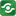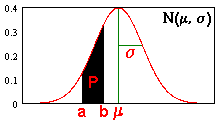Probability Z-Distribution@import url(http://www.google.com/cse/api/branding.css);CSGNetwork Custom SearchCSGNetwork Snippets Calculators Top Free Apps Home Back

Right click this window and select "view source" in order to copy the source for this script.

# Probability Z-Distribution

To find the area P under the normal probability curve N(mean, standard_deviation) within the interval (left, right), type in the 4 parameters and press "Calculate". The standard normal curve N (0,1) has a mean=0 and s.d.=1. Use -inf and +inf for infinite limits.

 left bound right bound mean standard deviation